×#### Thank you for registering.

One of our academic counsellors will contact you within 1 working day.

Click to Chat

1800-1023-196

+91-120-4616500

CART 0

• 0

MY CART (5)

Use Coupon: CART20 and get 20% off on all online Study Material

ITEM
DETAILS
MRP
DISCOUNT
FINAL PRICE
Total Price: Rs.

There are no items in this cart.
Continue Shopping• Complete JEE Main/Advanced Course and Test Series
• OFFERED PRICE: Rs. 15,900
• View Details

Revision Notes on Surface Areas and Volumes

Surface Areas and Volumes

Surface Area is the area of the outer part of any 3D figure and Volume is the capacity of the figure i.e. the space inside the solid. To find the surface areas and volumes of the combination of solids, we must know the surface area and volume of the solids separately. Some of the formulas of solids are -

Name
Figure
Lateral or Curved Surface Area
Total Surface Area
Volume
Length of diagonal and nomenclature

Cube4l2
6l2
l3

√3

l = edge of the cube

Cuboid2h(l +b)
2(lb + bh + hl)
lbh

l = length

h = height

Cylinder2πrh
2πr2 + 2πh = 2πr(r + h)
πr2h

h = height

Hollow cylinder2πh (R + r)
2πh (R + r) + 2πh (R2 - r2)
-

Cone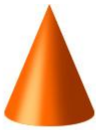πr2 + πrl = πr(r + l)
1/3 πr2h

h = height

l = slant height

Sphere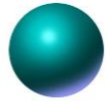4πr2
4πr2
4/3 πr3

Hemisphere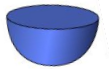2πr2
3πr2
2/3 πr3

Spherical shell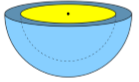4πR2 (Surface area of outer)
4πr2 (Surface area of outer)
4/3 π(R3 – r3)

PrismPerimeter of base × height
Lateteral surface area + 2(Area of the end surface)
Area of base × height
-

pyramid1/2 (Perimeter of base) × slant height
Lateral surface area + Area of the base
1/3 area of base × height
-

Surface Area of a Combination of Solids

If a solid is molded by two or more than two solids then we need to divide it in separate solids to calculate its surface area.

Example

Find the total surface area of the given figure.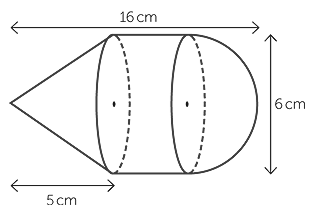Solution

This solid is the combination of three solids i.e.cone, cylinder and hemisphere.

Total surface area of the solid = Curved surface area of cone + Curved surface area of cylinder + Curved surface area of hemisphere

Curved surface area of cone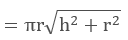Given, h = 5cm, r = 3cm (half of the diameter of hemisphere)

Curved surface area of cylinder = 2πrh

Given, h = 8cm (Total height – height of cone – height of hemisphere), r = 3cm

Curved surface area of hemisphere = 2πr2

Given, r = 3 cm

Total surface area of the solidVolume of a combination of solids

Find the volume of the given solid.Solution

The given solid is made up of two solids i.e. Pyramid and cuboid.

Total volume of the solid = Volume of pyramid + Volume of cuboid

Volume of pyramid = 1/3 Area of base x height

Given, height = 6 in. and length of side = 4 in.

Volume of cuboid = lbh

Given, l = 4 in., b = 4 in, h = 5 in.

Total volume of the solid = 1/3 Area of base x height + lbh

= 1/3 x 4 x 4 x 6 + (4) (4) (5)

= 32 + 80

= 112 in3

Conversion of Solid from One Shape to Another

When we convert a solid of any shape into another shape by melting or remoulding then the volume of the solid remains the same even after the conversion of shape.

Example

If we transfer the water from a cuboid-shaped container of 20 m x 22 m into a cylindrical container having a diameter of 2 m and height of 3.5 m. then what will be the height of the water level in the cuboid container if the cylindrical tank gets filled after transferring the water.Solution

We know that the volume of the cuboid is equal to the volume of the cylinder.

Volume of cuboid = volume of cylinder

l x b x h = πr2h

20 x 22 x h = 22/7 x 1 x 3.5

440 × h =11

H = 2.5 cm

Frustum of a Cone

If we cut the cone with a plane which is parallel to its base and remove the cone then the remaining piece will be the Frustum of a Cone.Volume of the frustum of the cone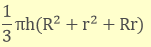The curved or Lateral surface area of the frustum of the cone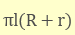Total surface area of the frustum of the cone
Area of the base + Area of the top + Lateral surface area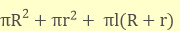Slant height of the frustum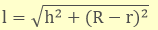Example

Find the lateral surface area of the given frustum of a right circular cone.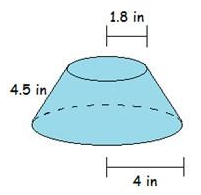Solution

Given, r =1.8 in.

R = 4 in.

l = 4.5 in.

The lateral surface area of the frustum of the cone = πl (R + r)

= π x 4.5 (4 +1.8)

=3.14 x 4.5 x 5.8

= 81.95 sq. in.### Course Features

• 728 Video Lectures
• Revision Notes
• Previous Year Papers
• Mind Map
• Study Planner
• NCERT Solutions
• Discussion Forum
• Test paper with Video Solution